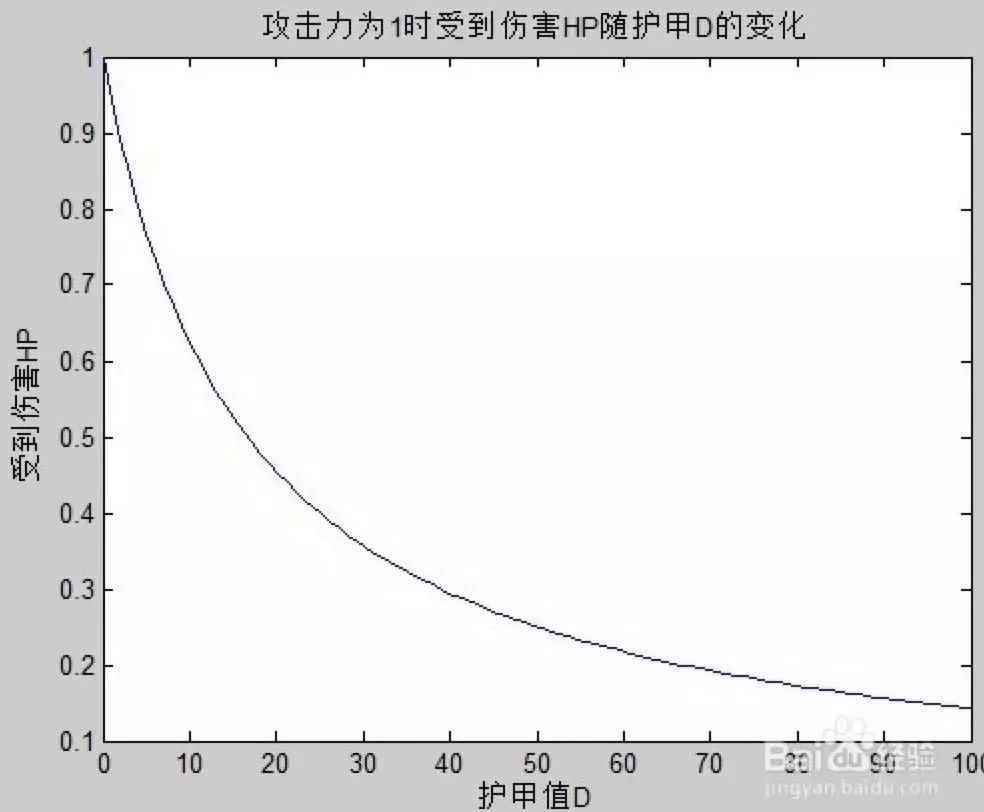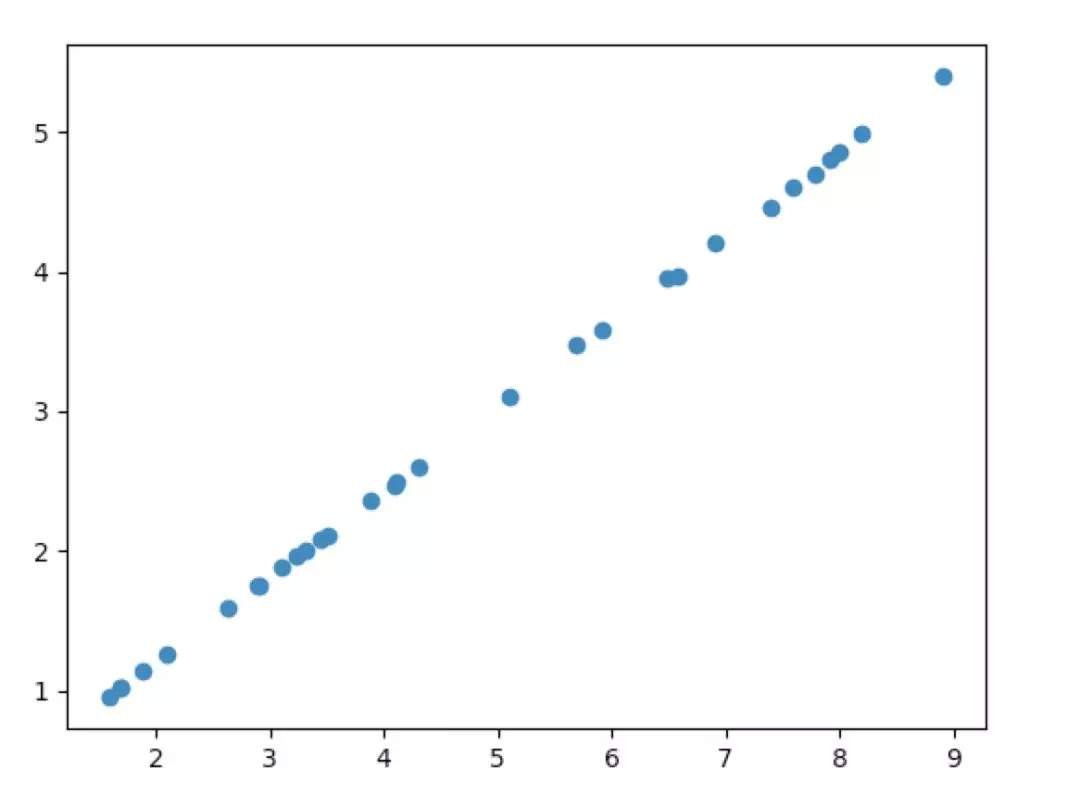# 以王者农药为例，入 tensorflow 之门## 正文python  import tensorflow as tf  import matplotlib.pyplot as plt  import numpy as np 

1，numpy 一个数组处理库。

2，tensorflow 我们今天的主角。

3，matplotlib 绘图库用来最后验证结论。

python # 再看这里先定义两个猜测存在的变量 w、b，tf.Variable([初始值], dtype= 变量类型)，Variable 就是 tf 中的定义变量的封装了 w = tf.Variable([1.0], dtype=tf.float64) b = tf.Variable([0.0], dtype=tf.float64) # 最后看这里我们再定义两个变量 x（攻击）、y（伤害），因为我们这个地方还没导入数据，但是又要提前定义，所以 tf 为我们贴心的设计了 placeholder 用于解决这种情况 x = tf.placeholder(tf.float64) y = tf.placeholder(tf.float64) # 先看这里，我们先假设最终的答案是 伤害 = 攻击力 * w + b 其中 w 和 b 是我们猜测可能存在的影响变量  linear_model = x * w + b

python #training & testing data atk_train = np.array( [289., 389., 409., 569., 649., 659., 739., 759., 779., 799., 819., 169., 264., 291., 311., 324., 331., 344., 351., 411., 431., 511., 591., 691., 791., 209.0]).astype(np.float32) / 100  damage_train = np.array( [175., 236., 247., 348., 395., 397., 446., 460., 470., 486., 499., 102., 160., 175., 189., 196., 201., 208., 211., 250., 260., 310., 358., 420., 480., 126.0]).astype(np.float32) / 100  atk_test = np.array([159.0, 169.0, 189.0, 891.]).astype(np.float32) / 100 damage_test = np.array([96.0, 102.0, 114.0, 540.]).astype(np.float32) / 100 

python #loss function loss = tf.reduce_sum(tf.square(linear_model - y)) #optimizer optimizer = tf.train.GradientDescentOptimizer(0.001)  train = optimizer.minimize(loss) #accuracy correct_prediction = tf.abs(tf.cast(linear_model, tf.int32) - tf.cast(y, tf.int32)) < 2  accuracy = tf.reduce_mean(tf.cast(correct_prediction, tf.float32)) 

tf.reduce_sum(tf.square(linear_model - y)) 是什么意思呢，linear_model 是我们预测的答案，y 是标准答案。我们用 (linear_model - y) 的平方来表示是否最接近标准答案，很明显 0 是最完美的情况。然后 reduce_sum 是什么意思呢，是分别拿所有的 training data 的最终反馈一一相加。这就是我们定义的 loss function。

optimizer 是优化器，我们已经定义好了 model 和 loss，怎么样去优化参数呢，就需要一个优化器就是 optimizer，这个地方直接使用 tf 已经封装好的 tf.train.GradientDescentOptimizer(0.001)，这个优化器用到的算法是梯度下降。这个算法是怎么来的，又有哪些问题，教程很多这里就略过不讲了，目标就就是知其然。

python #初始化所有变量 init = tf.global_variables_initializer() #训练的时候，tf 规定必须运行在一个 session 里。所以我们 new 一个 session sess = tf.Session() 并且初始化他 sess.run(init) #开始训练，循环 1W 次 for i in range(100000): # 传入要训练的 train, 和需要的训练数据      data = sess.run(train, {x: atk_train, y: damage_train})

python #evaluate training accuracy curr_w, curr_b, curr_loss, curr_accuracy = sess.run([w, b, loss, accuracy], {x: atk_train, y: damage_train})  print("w: %s b: %s loss: %s accuracy: %s" % (curr_w, curr_b, curr_loss, curr_accuracy))   curr_w, curr_b, curr_loss, curr_accuracy = sess.run([w, b, loss, accuracy], {x: atk_test, y: damage_test})  print("w: %s b: %s loss: %s accuracy: %s" % (curr_w, curr_b, curr_loss, curr_accuracy))

output: w: [ 0.6076637] b: [-0.00811569] loss: 0.00450731719102 accuracy: 1.0 w: [ 0.6076637] b: [-0.00811569] loss: 4.32571875675e-05 accuracy: 1.0

## 后记

python  import tensorflow as tf  import matplotlib.pyplot as plt  import numpy as np #Model parameters w = tf.Variable([1.0], dtype=tf.float64)  b = tf.Variable([0.0], dtype=tf.float64) #Model input and output x = tf.placeholder(tf.float64)  y = tf.placeholder(tf.float64) linear_model = x * w + b #training data atk_train = np.array( [289., 389., 409., 569., 649., 659., 739., 759., 779., 799., 819., 169., 264., 291., 311., 324., 331., 344., 351., 411., 431., 511., 591., 691., 791., 209.0]).astype(np.float32) / 100  damage_train = np.array( [175., 236., 247., 348., 395., 397., 446., 460., 470., 486., 499., 102., 160., 175., 189., 196., 201., 208., 211., 250., 260., 310., 358., 420., 480., 126.0]).astype(np.float32) / 100  atk_test = np.array([159.0, 169.0, 189.0, 891.]).astype(np.float32) / 100  damage_test = np.array([96.0, 102.0, 114.0, 540.]).astype(np.float32) / 100 #loss function loss = tf.reduce_sum(tf.square(linear_model - y)) #optimizer optimizer = tf.train.GradientDescentOptimizer(0.001)  train = optimizer.minimize(loss) #accuracy correct_prediction = tf.abs(tf.cast(linear_model, tf.int32) - tf.cast(y, tf.int32)) < 2  accuracy = tf.reduce_mean(tf.cast(correct_prediction, tf.float32)) #training loop init = tf.global_variables_initializer()  sess = tf.Session() sess.run(init)  for i in range(100000):      data = sess.run(train, {x: atk_train, y: damage_train}) #evaluate training accuracy curr_w, curr_b, curr_loss, curr_accuracy = sess.run([w, b, loss, accuracy], {x: atk_train, y: damage_train})  print("w: %s b: %s loss: %s accuracy: %s" % (curr_w, curr_b, curr_loss, curr_accuracy))   curr_w, curr_b, curr_loss, curr_accuracy = sess.run([w, b, loss, accuracy], {x: atk_test, y: damage_test})  print("w: %s b: %s loss: %s accuracy: %s" % (curr_w, curr_b, curr_loss, curr_accuracy)) 

https://mp.weixin.qq.com/s/d14Qip_NN2eWAelLpNViKg